# Normal Force and Friction Force Normal Force is

• Slides: 21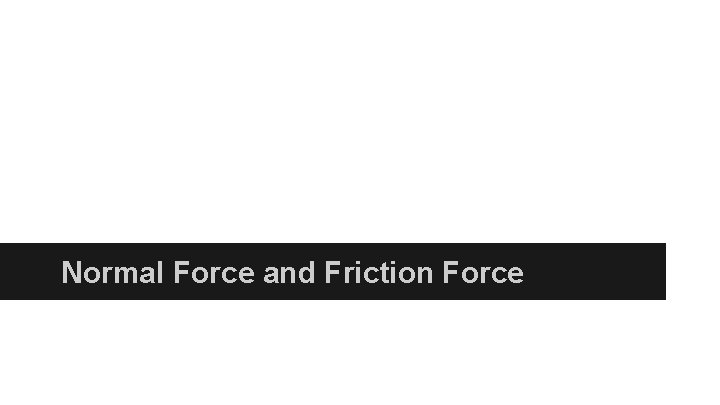Normal Force and Friction ForceNormal Force is always perpendicular to the surface that an object is on.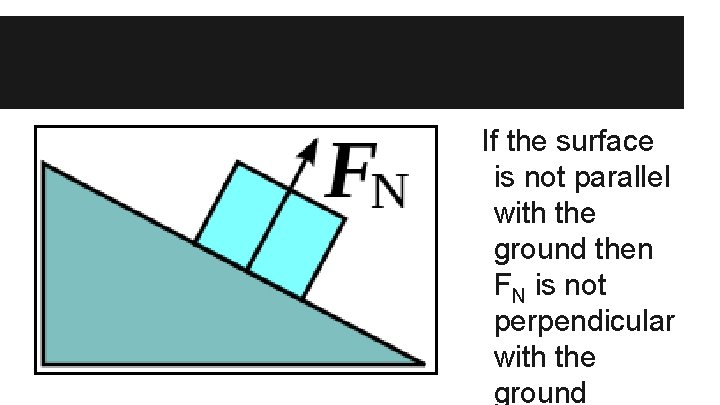If the surface is not parallel with the ground then FN is not perpendicular with the ground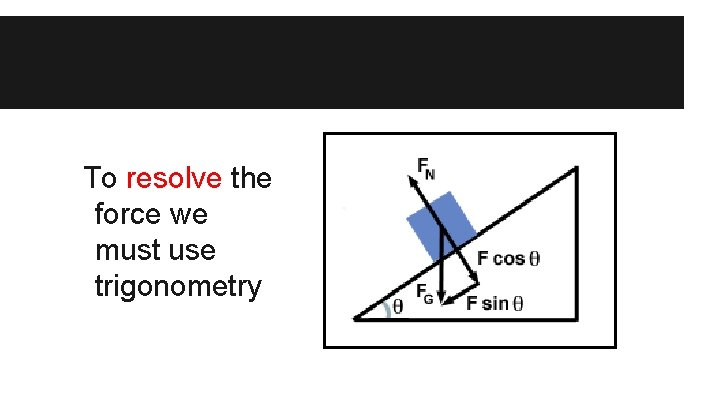To resolve the force we must use trigonometryOnce resolved we only use the forces that are perpendicular to each other in solving problemscan ignore now Fg is no longer useful as we now know its vector components (Fgx and Fgy)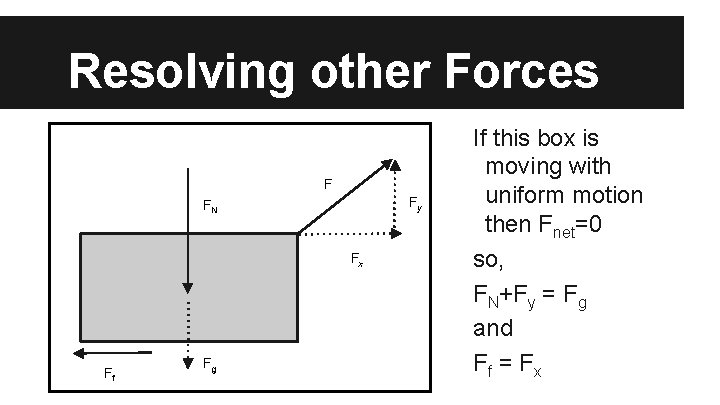Resolving other Forces F Fy FN Fx Ff Fg If this box is moving with uniform motion then Fnet=0 so, FN+Fy = Fg and Ff = F x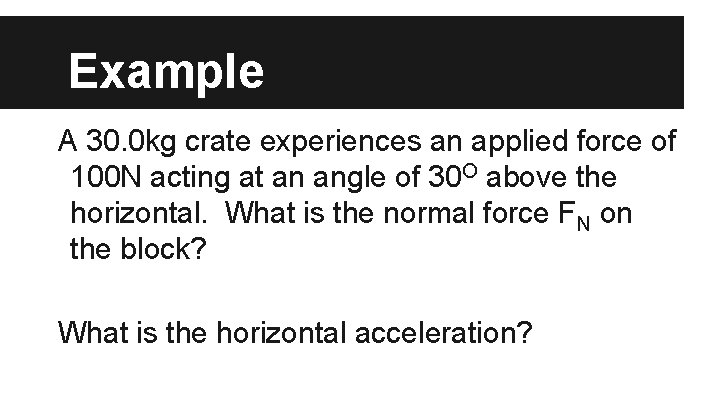Example A 30. 0 kg crate experiences an applied force of 100 N acting at an angle of 30 O above the horizontal. What is the normal force FN on the block? What is the horizontal acceleration?Friction A force that oppose two objects sliding against one another. Two types a) kinetic friction b) static frictionKinetic Friction (fk) The frictional force that occurs between two objects sliding against each other. 3 basic rules 1. fk is independent of velocity 2. fk is independent of contact surface area 3. fk is directly proportional to FN and how ‘rough’ the surfaces areTo find kinetic friction (fk) fk = k FN coefficient of friction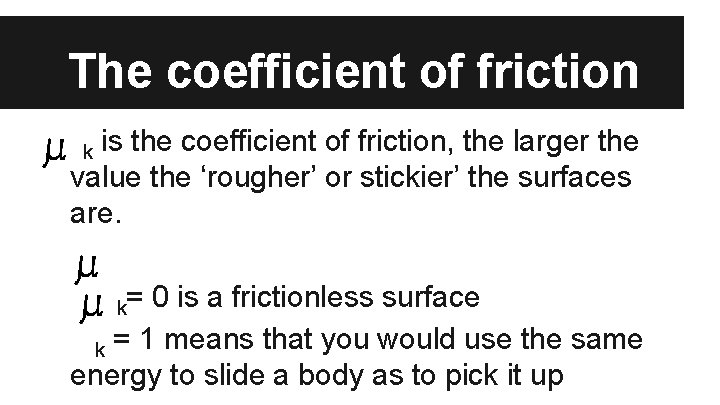The coefficient of friction is the coefficient of friction, the larger the value the ‘rougher’ or stickier’ the surfaces are. k k= 0 is a frictionless surface k = 1 means that you would use the same energy to slide a body as to pick it upExample 1 A 20 kg sled slides over a horizontal icy surface against a frictional force of 16 N. Find the Coefficient of kinetic friction between the sled and the ice.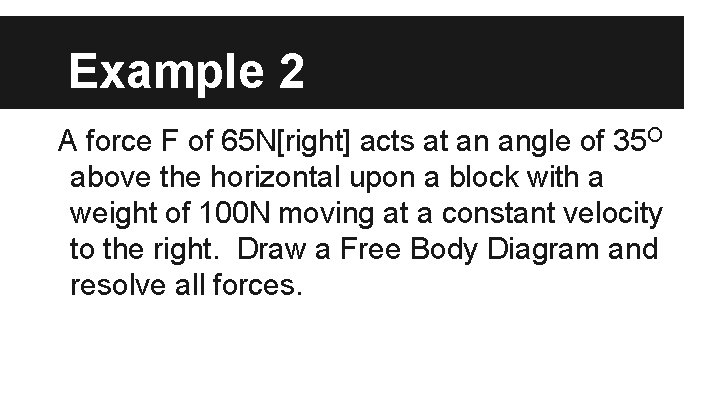Example 2 A force F of 65 N[right] acts at an angle of 35 O above the horizontal upon a block with a weight of 100 N moving at a constant velocity to the right. Draw a Free Body Diagram and resolve all forces.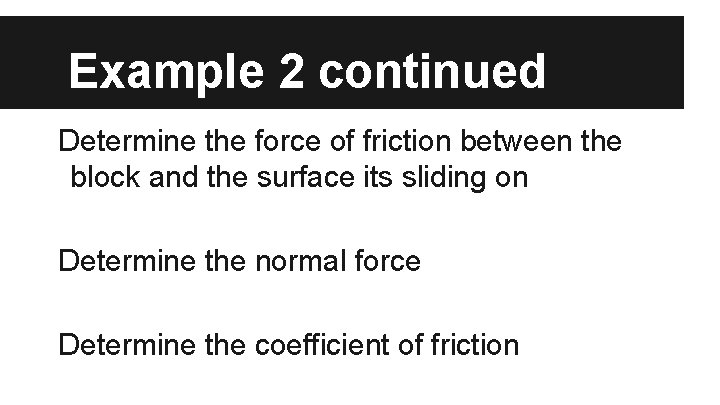Example 2 continued Determine the force of friction between the block and the surface its sliding on Determine the normal force Determine the coefficient of frictionStatic Friction fs Static friction exists when the contact surfaces do not slide relative to one another. objects s > k, there is less friction when are moving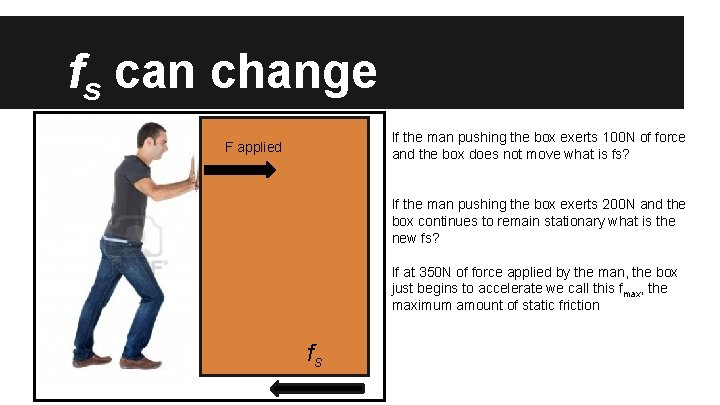fs can change If the man pushing the box exerts 100 N of force and the box does not move what is fs? F applied If the man pushing the box exerts 200 N and the box continues to remain stationary what is the new fs? If at 350 N of force applied by the man, the box just begins to accelerate we call this fmax, the maximum amount of static friction fs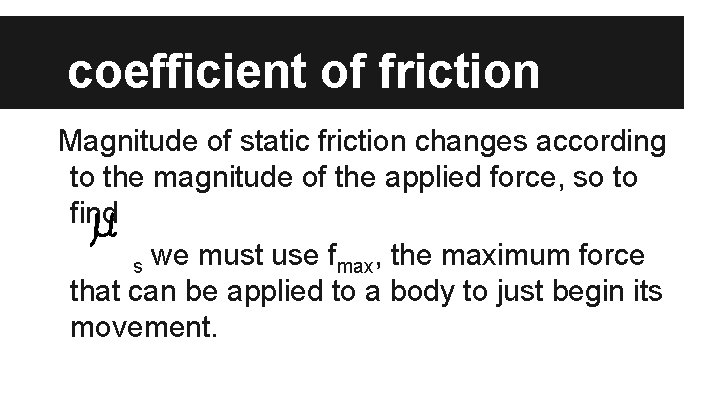coefficient of friction Magnitude of static friction changes according to the magnitude of the applied force, so to find s we must use fmax, the maximum force that can be applied to a body to just begin its movement.Example A man is pushing a couch and it just begins to move when a force of 150 N is applied to it. What is the fmax between the couch and floor? What is the coefficient of friction if the couch weighs 100 kg?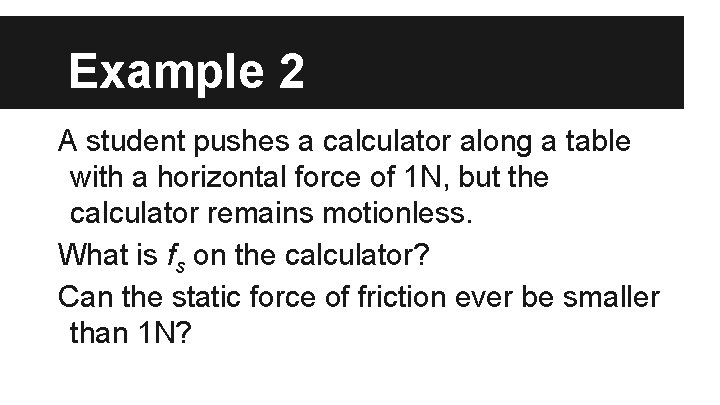Example 2 A student pushes a calculator along a table with a horizontal force of 1 N, but the calculator remains motionless. What is fs on the calculator? Can the static force of friction ever be smaller than 1 N?Example 3 A woman pulls on a 30 kg box with a rope at an angle at 25 O. She initially pulls with a force of 90 N without moving the box 30 kg